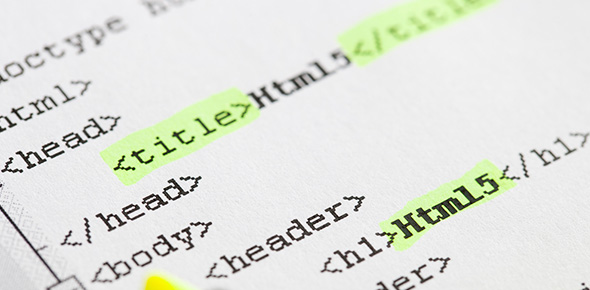# Independent And Dependent Variables Quiz

15 Questions | Total Attempts: 2371SettingsIndependent and dependent variables

• 1.
If you chew 2 different flavors of gum which flavor will last longer? What is the independent variable?
• A.

Cake

• B.

Flavor of gum

• C.

How long gum lasts

• D.

None of the above

• 2.
If we add weight to a helicopter, will it stay in the air longer? What is the dependent variable?
• A.

Amount of time in the air

• B.

Weight

• C.

How big the helicopter is

• D.

The color of the helicopter

• 3.
What is the dependent variable in this question: If we make the walls of a boat higher, will it float longer?
• A.

What is in the boat

• B.

Depth of water

• C.

Height of walls

• D.

How long it floats

• 4.
What is the independent variable? If we add more icing to a cake, will it produce mold faster?
• A.

Type of icing

• B.

Number of people

• C.

Amount of icing

• D.

Number of cakes

• 5.
The variable that you measure, is the:
• A.

Independent Variable

• B.

Dependent Variable

• C.

Controlled Variable

• D.

Repeated Trial

• 6.
The independent variable is the ONE thing you...
• A.

Change

• B.

Keep the same

• C.

Experiment with

• D.

Investigate

• 7.
How many independent variables can be tested in a controlled experiment?
• A.

One

• B.

Two

• C.

Three

• D.

As many as possible

• 8.
Miss Snooks set up an experiment to see how the mass of a ball affects the distance it rolls off a ramp. What is the dependent variable?
• A.

Mass of the ball

• B.

Height of the ramp

• C.

Distance traveled by the ball.

• 9.
Justin is planning an experiment to find out how the height from which he drops a ball affects how high the ball bounces. What is the control variable?
• A.

The height the ball bounces

• B.

The ball used

• C.

Height of the ball being dropped

• 10.
How does watching TV while studying affect a students mark? Which variable is highlighted in yellow?
• A.

Independent variable

• B.

Dependent variable

• C.

Controlled variable

• 11.
What effect does decreasing the temperature of a room have on the life of a battery? Which variable is highlighted in yellow?
• A.

Independent variable

• B.

Dependent variable

• C.

Controlled variable

• 12.
The measurable factor in an experiment is known as the:
• A.

Independent variable

• B.

Dependent variable

• C.

Control Variable

• D.

Constant variable

• 13.
The variable that I change, is the?
• A.

Control variable

• B.

Dependent variable

• C.

Independent variable

• 14.
A variable that depends on what I have changed, is the?
• A.

Controlled variable

• B.

Independent variable

• C.

Dependent variable

• 15.
A variable that we keep the same?
• A.

Control variable

• B.

Independent variable

• C.

Dependent variable

Related TopicsBack to top